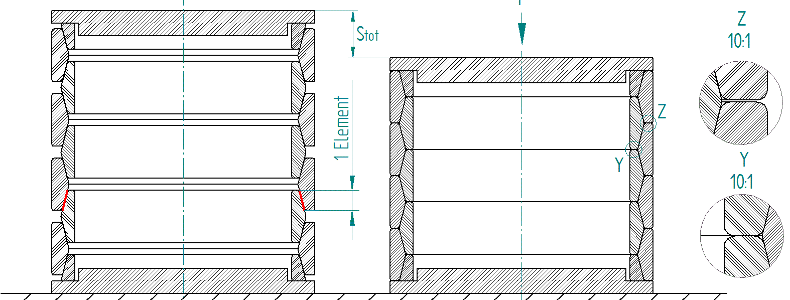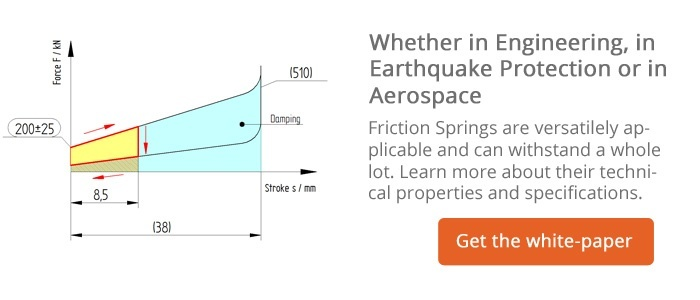# How does a Friction Spring Work?

In a previous article we covered friction springs and their applications. This time we would like to explain the technical properties of a friction spring, how it works and why its features provide a variety of advantages compared to other damping systems.

#### Composition of a friction spring

Friction springs consist of precisely manufactured outer and inner rings which touch each other on their tapered faces. This contact surface is called element. Figure 1 shows a friction spring that is composed of four outer rings, three inner rings and two inner ring halves, i.e. the friction spring comprises 8 elements.Fig. 1: Configuration of a friction spring with 8 elements, at rest (left) and loaded (right)

The number of elements is essential for the design of the spring because it determines the total travel and the total spring work. A friction spring works best when it ends with inner ring halves. For this reason, an even number of elements e is preferably selected.

In some cases, however, the size of the friction spring is determined on the basis of the available installation space. If the spring then consists of an uneven number of elements, it ends with one half an inner ring and one half an outer ring.

The selection of the ring size defines further essential parameters for a friction spring:

• The end force F,
• The outer and inner guide diameters D2 and d2,
• The element height he,
• The spring travel per element se
• The energy absorption per element We.

For a given application, the required number of elements e is calculated from the desired spring travel or from the required spring work W, while the pre-load is neglected. Finally, the individual rings are built up to form complete spring columns.

#### How a friction spring works

When an axial load is imposed on the spring column, the tapered faces are caused to slide against one another and the individual rings are deformed elastically (Fig. 1). Eventually, this means: The outer rings are stretched and the inner rings are compressed. The resulting spring travel se is calculated from the change in diameter of the elastically deformed rings and their angle of taper.

Accordingly, the total spring travel of a friction spring is the product of the number of elements e and the spring travel se, whereby the spring force does not change with the number of elements.

In contrast to other spring types, the circumferential stresses are almost uniformly distributed over the cross-sectional area of the loaded spring elements. Therefore, each volume element is utilized uniformly. This helps reduce the weight of the friction spring compared to other conventional spring types, where the cross-section is dimensioned according to the maximum edge stresses, while the neutral axis (core) remains unloaded.

Typically, a spring is designed for a utilization of about 70 % so that its beneficial properties are combined for a service life as long as possible. To ensure its operational reliability, each spring is loaded twice to 140 % of its end force.

#### Properties of friction springs

• High spring work with low weight and volume
• Characteristic curve can be varied by the selection of the element number
• Linear characteristic curve
• Overload proof when in blocked (solid) position
• High damping capability
• Force-travel curve independent of the loading rate
• Curve independent of the temperature
• Reproducibility of the characteristic curve
• Maintenance-free
• Variable configuration of the friction spring
• Parallel arrangement for higher forces and serial arrangement for greater spring travels possible

The friction spring is superior to many other damping systems and is suited for use in a variety of applications. One particular and important application area for which friction springs are notably suitable is the field of earthquake protection. More details about the use of friction springs in earthquake protection will follow in one of our next blog articles.